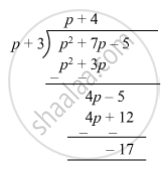SSC (English Medium) Class 8Maharashtra State Board
Share

# Divide and Write the Quotient and the Remainder. (P2 + 7p − 5) ÷ (P + 3) - SSC (English Medium) Class 8 - Mathematics

ConceptTo Divide a Polynomial by a Binomial

#### Question

Divide and write the quotient and the remainder.

(p2 + 7p − 5) ÷ (p + 3)

#### Solution

(p2 + 7p − 5) ÷ (p + 3)So, quotient = p + 6 and remainder = $-$ 17 .

Is there an error in this question or solution?

#### APPEARS IN

Balbharati Solution for Balbharati Class 8 Mathematics (2019 to Current)
Chapter 10: Division of Polynomials
Practice Set 10.2 | Q: 2 | Page no. 66
Solution Divide and Write the Quotient and the Remainder. (P2 + 7p − 5) ÷ (P + 3) Concept: To Divide a Polynomial by a Binomial.
S